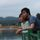cancel
Showing results for
Did you mean:Helper V

## DAX Count of Stores that are under the Average

Hi there,

I am struggleing with a DAX Measure, I would like to count the number of Stores that are under the average sales amount.

I am the following supporting DAX Measures.

This Year To Date Units

`TYTD Units = TOTALYTD(CALCULATE([Total Units]),'CALENDAR'[DATE])`

Number of Unique Stores/Sites

`Site Count = CALCULATE(DISTINCTCOUNT(MASTER_SITE[STORE NAME]))`

The average Unit Sales (This Year To Date)

`TYTD Store Average (U) = DIVIDE([TYTD Units],[Site Count],0)`

So the result I am looking for is the distinct count of Stores/Sites that are under the average Unit sales.

3 REPLIES 3Memorable Member

Hi @MarkCBB

Your concern is really really interesting. I will create one Dates table and 3 calculated measure:

• This Year to date Units

`TYTD Units = CALCULATE(SUM(Trans[Unit]),filter(all(Dates[Date]),Dates[Date] <= MAX(Dates[Date]) ))`• The average unit Sales (This Year To Date)

`TYTD Store Average (U) = CALCULATE(DIVIDE([TYTD Units],DISTINCTCOUNT(Trans[Store])),ALLEXCEPT(Trans,Dates[Date]))`

Re-use YTD units and using ALLEXCEPT(Date) to ensure that same date will have same average sales value. Please take a look top right table:• The rest of things is count store/site under average:

`No. of Store/Sites under AVG = CALCULATE( DISTINCTCOUNT(Trans[Store]),filter(Trans,[TYTD Units] < [TYTD Store Average (U)] ))`

If this works for you please accept it as solution and also like to give KUDOS.Helper V

Hello @tringuyenminh92

Thank you for your detailed responce, I have been able to implement this, however the solution you have given is very heavy on RAM. I believe that it is the FILTER() that is using so much, my fact table is just over 10million rows. I have 64g RAM (1600MHZ). And I used 100%. I have to use filters (i.e. Region.Proviance) to get the result to calculate.

Can you think of another way to do this that does not use FILTER. Or maybe a New table to cut down the number of FILTERS that run.

PS, I remove the Date MAX, from the Measure as I am already using the TOTALYTD, with a Calendar Table.

I look forward to your responce.

Regards,

MarkCBB.Memorable Member

Hi @MarkCBB,

I will say Yes, we could consider and prefer using Calculated Column than Calculated Measure (to cut down memory computing), but when using Calculated Column, we need to handle row context and this will 2 two risks as my perspective:

• if we have some filter by another Dims like times, stores or region/province,... (even though we could use allexcept, allselected for filters situation)
• if we support multiple select in slicers, we might not handle it by DAX

So i will replace measure TYTD Units by calculated column:

`TYTD Units - By Calculated Column = CALCULATE(SUM(Trans[Unit]),filter(ALLEXCEPT(Trans,Trans[Store]),Trans[Date] <= EARLIER('Trans'[Date])))`

and use it in TYTD Store Average (U) :

`TYTD Store Average (U) - using TYTD column = CALCULATE(DIVIDE(sum(Trans[TYTD Units - By Calculated Column]),DISTINCTCOUNT(Trans[Store])),ALLEXCEPT(Trans,Dates[Date]))`

You could try to make "TYTD Store Average (U)" or "No. of stores under average" by calculated column, but i know you will show them in lots of charts and tables with slicers so there are a lot of things need to be done when doing that. it's really complicated now 😞Announcements#### Exclusive opportunity for Women!

Join us for a free, hands-on Microsoft workshop led by women trainers for women where you will learn how to build a Dashboard in a Day!#### Power Platform Conference-Power BI and Fabric Sessions

Join us Oct 1 - 6 in Las Vegas for the Microsoft Power Platform Conference.Top Solution Authors
Top Kudoed Authors
Users online (3,125)